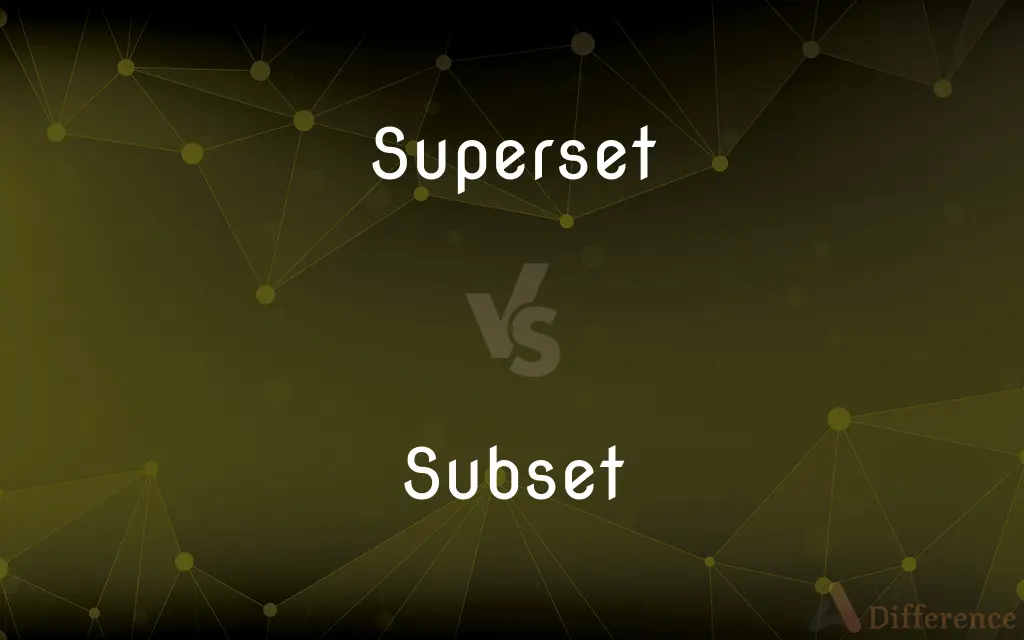# Superset vs. Subset — What's the Difference?## Definitions

#### Superset➦

(set theory) (symbol: ⊇) With respect to another set, a set such that each of the elements of the other set is also an element of the set.
The set of human beings is a superset of the set of human children.
The set of characters "LBPG" is a superset of the set of characters "PG".

#### Subset➦

In mathematics, a set A is a subset of a set B if all elements of A are also elements of B; B is then a superset of A. It is possible for A and B to be equal; if they are unequal, then A is a proper subset of B. The relationship of one set being a subset of another is called inclusion (or sometimes containment). A is a subset of B may also be expressed as B includes (or contains) A or A is included (or contained) in B. The subset relation defines a partial order on sets.

#### Superset➦

(weightlifting) Two or more different physical exercises performed back to back, without a period of rest between them. The exercises may employ the same muscle group, or opposing muscle groups.

#### Subset➦

A set contained within a set.

#### Superset➦

To perform (different physical exercises) back to back, without a period of rest between them.

#### Subset➦

A set A such that every element of A is also an element of S.
The set of integers is a subset of the set of real numbers.
The set $\lbrace a, b\rbrace$ is a both a subset and a proper subset of $\lbrace a, b, c\rbrace$ while the set $\lbrace a, b, c\rbrace$ is a subset of $\lbrace a, b, c\rbrace$ but not a proper subset of $\lbrace a, b, c\rbrace$.

#### Subset➦

A group of things or people, all of which are in a specified larger group.
We asked a subset of the population of the town for their opinion.

#### Subset➦

(transitive) To take a subset of.

#### Subset➦

To extract only the portions of (a font) that are needed to display a particular document.

#### Subset➦

A set whose members are members of another set; a set contained within another set